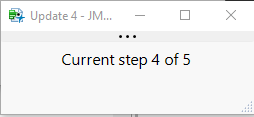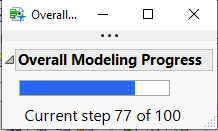Choose Language Hide Translation Bar
Highlighted

## Suggestion on how to script a small update/progress window for modeling

Dear JMP Community,

I'm interested in scripting a small window that pops up when I run my model building script to show me at which step (out of thousands) is it currently running. I'm thinking of doing something like the following script, which works, but also makes a new window each time.

``````Names Default To Here( 1 );
imax = 5;
For( i = 1, i <= imax, i++,
update_window = New Window( "Progress Update",
Text Box( " Current step " || Char( i ) || " of " || Char( imax ), <<Set Font Size( 12 ), <<Justify Text( "center" ), <<Set width ( 250 ) ),

)
);``````

Of course, my imax is much larger than 5, this is just a test script. I get an output at each iteration of the following (five windows in this case):But, as mentioned, this would make several thousand such windows, which I don't want. I'd like to update just the step "i" of the total.

The modeling script is built into a data table and has it's own FOR loop as it's running a giant tuning table one line at a time -- imax is the NRows() of this tuning table, and can be on the order of 24k+ rows. I want to insert into the main for loop a script that creates a single window that stays on the screen and tells the user what step "i" it's at out of NRows(). (Scrolling through the data table is tedious, and this seems like a reasonable solution.)

I don't need a progress bar, or anything like that, just a simple number that changes at each iteration of the main FOR loop. There is only one FOR loop, BTW.

DS

1 ACCEPTED SOLUTION

Accepted Solutions
Highlighted

## Re: Suggestion on how to script a small update/progress window for modeling

Open the window with the update separately and before the iteration begins. Save a reference to the text box in a variable. Send the << Set Text( "new text" ) message to the reference inside the iterations.

``````Names Default To Here( 1 );
imax = 5;
update_window = New Window( "Progress Update",
tb = Text Box( "Initial message", <<Set Font Size( 12 ), <<Justify Text( "center" ), <<Set width ( 250 ) ),

);
For( i = 1, i <= imax, i++,
tb << Set Text( " Current step " || Char( i ) || " of " || Char( imax ) );
);``````
Learn it once, use it forever!
3 REPLIES 3
Highlighted

## Re: Suggestion on how to script a small update/progress window for modeling

Open the window with the update separately and before the iteration begins. Save a reference to the text box in a variable. Send the << Set Text( "new text" ) message to the reference inside the iterations.

``````Names Default To Here( 1 );
imax = 5;
update_window = New Window( "Progress Update",
tb = Text Box( "Initial message", <<Set Font Size( 12 ), <<Justify Text( "center" ), <<Set width ( 250 ) ),

);
For( i = 1, i <= imax, i++,
tb << Set Text( " Current step " || Char( i ) || " of " || Char( imax ) );
);``````
Learn it once, use it forever!
Highlighted

## Re: Suggestion on how to script a small update/progress window for modeling

Hi @markbailey,

I was moving in this direction after reading through this post: https://community.jmp.com/t5/Discussions/JMP-script-progress-indicator/m-p/6057#M6056. But, I was still a few steps away. For the test script, I needed to add in a Wait() in the FOR loop because it went so fast. The modeling takes longer at each step, so I am not worried about this.

In future version of this, I might change it to a "progress bar" by modifying what the other user in the above post did.

Thanks again!,

DS

Highlighted

## Re: Suggestion on how to script a small update/progress window for modeling

Update:

After modifying the JSL in the post that I referenced, I was able to create this test window to show the progress as a bar (visual), plus the actual step count X of Y. This was fun!

``````Names Default To Here( 1 );
i = 1;
imax = 100;
dlgStatus = New Window( "Overall Modeling Progress",
V List Box(
dlg_gb = Graph Box(

Title( "Overall Modeling Progress" ),

FrameSize( 150, 15 ),

X Scale( 0, 100 ),

Y Scale( 0, 1 ),

yaxis( show major ticks( 0 ), show minor ticks( 0 ) ),

xname( "% complete" ),

yname( "" )

),
tb = Text Box(
" Current step " || Char( i ) || " of " || Char( imax ),
<<Set Font Size( 12 ),
<<Justify Text( "center" ),
<<Set width( 200 )
)
)
);

dlg_gb[Axis Box( 2 )] << Delete;
dlg_gb[Axis Box( 1 )] << Delete;

For( i = 1, i <= imax, i++,
prog = (i / imax) * 100;
dlgStatus[FrameBox( 1 )] << Add Graphics Script( {Fill Color( "blue" ), Rect( 0, 1, prog, 0, 1 )} );
tb << set text( " Current step " || Char( i ) || " of " || Char( imax ) );
Wait( 0.1 );
);

dlgStatus << closewindow();``````Article Labels

There are no labels assigned to this post.